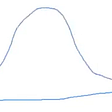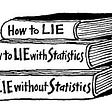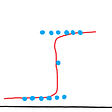# The acid test of a generative model for data.

A famous 2010 paper To Explain or to Predict? by Galit Shmueli examined the essential difference between classical mathematical statistics and modern machine learning. Amazon retail uses its data on you and others to predict what you might buy, without caring why you might want to buy it — an iconic use of machine learning. In contrast a classical statistician might use (say) a multivariate Normal model merely for analytic convenience. But an implicit, and sometimes explicit, aspect of a probability model is the suggestion of causality: that observed data on smoking and lung cancer not only shows an association between factor and effect within a probability model taking account of other potentially relevant factors, but also suggests that smoking is often a cause of lung cancer. This is the “to explain” side of the dichotomy.

In a simpler context, the fact that many types of observational numerical data show a rough fit to some power law distribution led in the early 2000s to much literature devising models that purport to explain how this occurs. But in fact many different mathematical models lead to approximate power law distributions — see the (also famous) 2004 paper A brief history of generative models for power law and lognormal distributions by Michael Mitzenmacher. So why should we believe a particular one?

Here’s the general issue. You have some data which exhibits some feature you find noteworthy. So you devise and study models until you find one which reproduces that feature. Then you shout Eureka! — this model explains how the feature arises in this data. But of course this procedure merely demonstrates your persistence in continuing to examine models until you found a good match to this one feature. The first one-armed man you find is likely not the real killer.

So consider a generative model designed to reproduce a given feature. How to make a step toward evidence of causality — to argue that the internal ingredients of the model can be matched with real world actions? To me, a basic acid test is

does your model also predict accurately a feature other than the one that you fitted?

Here is my favorite example. The random walk (or mathematical Brownian motion) model for stock index prices in the short term (up to a week, say) is generally regarded as a reasonable first approximation, outside of rare “black swan” events. There are many ways one can test this — for instance the model says the the variance of the price change over a time interval of length t should should be proportional to t. Or test how well the Black-Scholes formula for option prices matches the actual prices. Now these relate to actual financial transactions. Our acid test is look at some aspect of prices that one cannot readily speculate on. And one remarkable aspect of mathematical Brownian motion is the three arc sine laws described in the linked Wikipedia page. In our context, take the stock index price over a time interval (and scale as 1 unit time). The model predicts that each of the following 3 statistics of this process

T = proportion of time that price > starting price

L = last time that price crosses over starting price

W = time within interval at which price is maximum

has the same probability density function, the arc sine density in the figure.

So is this true for stock prices, as a good approximation? Well, that would make a good project for a starting Data Science student, partly because some initiative would be required to find the relevant non-standard data. I am confident that in fact one would find a quite good match, as in this old small-sample study. And that would be convincing evidence for the model, because there’s no way one can imagine this non-intuitive feature arising except by a calculation using the specific probability model.

## Conclusion

Be skeptical about any explanatory interpretation of a model designed to fit one aspect of data without examining other aspects.

--

--

--

## More from David Aldous

After a research career at U.C. Berkeley, now focussed on articulating critically what mathematical probability says about the real world.

Love podcasts or audiobooks? Learn on the go with our new app.

## Gaussians as Exponential Imposters and its Application in Quantum Chemistry## Why the rich will always become richer (unless they really screw it up)!## Repeated failure is the royal road to learning math## Type-2 Fuzzy Sets## Linear Algebra in Python## How to Randomize a Cube## David Aldous

After a research career at U.C. Berkeley, now focussed on articulating critically what mathematical probability says about the real world.

## Hypothesis Testing (t-tests)## Statistics and its Misapplications## Understanding the Cost function.## Solving Real-World problems using Logistic Regression